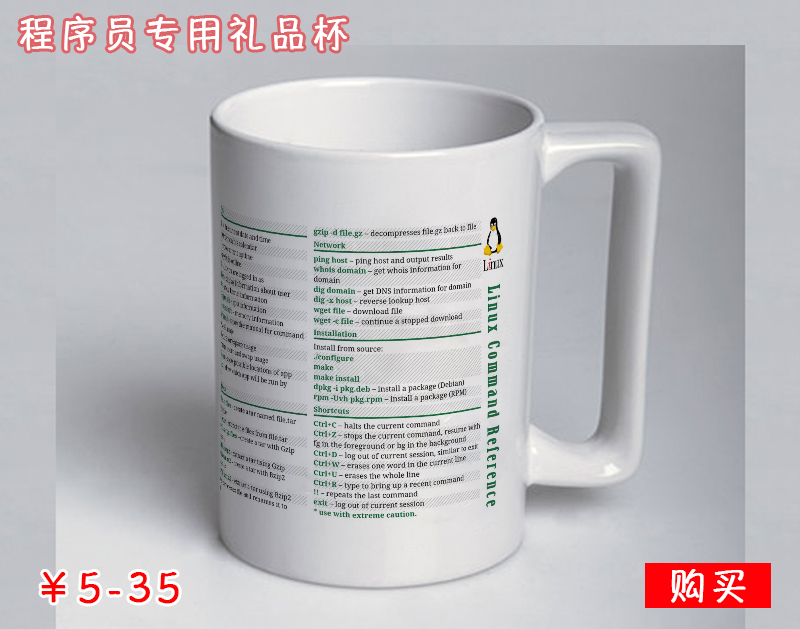## 1.将数组转化为列表

```List<String> list = Arrays.asList(arr);
```

`Arrays.asList()`会返回一个ArrayList对象，ArrayList类是Arrays的一个私有静态类，而不是java.util.ArrayList类，java.util.Arrays.ArrayList类有set()、get()、contains()方法，但是没有增加元素的方法，所以它的大小是固定的，想要创建一个真正的ArrayList类，你应该这样做:

```ArrayList<String> arrayList = new ArrayList<String>(Arrays.asList(arr));
```

ArrayList的构造方法可以接受一个集合类型，刚好它也是java.util.Arrays.ArrayList的超类。

## 2.判断一个数组是否包含一个值

```Set<String> set = new HashSet<String>(Arrays.asList(arr));
return set.contains(targetValue);
```

```Arrays.asList(arr).contains(targetValue);
```

```for(String s:arr){
if(s.equals(targetValue)){
return true;
}
}
return false;
```

## 3.在一个循环中删除一个列表中的元素

```ArrayList<String> list = new ArrayList<String>(Arrays.asList("a","b","c","d"));
for(int i=0;i<list.size();i++){
list.remove(i);
}
System.out.println(list);
```

```[b,d]
```

```ArrayList<String> list = new ArrayList<String>(Arrays.asList("a","b","c","d"));
for(String s:list){
if(s.equals("a")){
list.remove(s);
}
}
```

```ArrayList<String> list = new ArrayList<String>(Arrays.asList("a","b","c","d"));
Iterator<String> iter = list.iterator();
while(iter.hasNext()){
String s = iter.next();
if(s.equals("a")){
iter.remove();
}
}
```

`.next()`必须在`.remove()`之前调用。在一个foreach循环中，编译器会使.next()在删除元素之后被调用，因此就会抛出ConcurrentModificationException异常，你也许希望看一下ArrayList.iterator()的源代码。

## 5.在集合中使用原始类型

```public static void add(List list,Object o){
}
pulbic static void main(String[] args){
List<String> list = new ArrayList<String>();
String s = list.get(0);
```

```Exception in thread "main" java.lang.ClassCastException: java.lang.Integer cannot be cast to java.lang.String
at ...
```

## 8.可变与不可变不可变对象有许多的优点，比如简单，安全等等。但是对于每一个不同的值都需要一个独立的对象，太多的对象可能会造成大量的垃圾回收。当选择可变与不可变时应该有一个平衡。 一般的，可变对象用来避免产生大量的中间对象。一个典型的例子是连接大量的字符串。如果你用一个不可变的字符串，你会产生很多需要进行垃圾回收的对象。这很浪费CPU的时间，使用可变对象是正确的解决方案(比如StringBuilder)。

```String result="";
for(String s: arr){
result = result + s;
}
```

## 9.父类与子类的构造函数

```public Super(){
System.out.println("Super");
}
```

## 10.””还是构造器

```//1.使用字符串
String x = "abc";
//2.使用构造器
String y = new String("abc");
```

```String a = "abc";
String b = "abc";
System.out.println(a==b);//true
System.out.println(a.equals(b));//true

String c = new String("abc");
String d = new String("abc");
System.out.println(c==d);//false
System.out.println(c.equals(d));//true
```